# NCERT Exemplar Solutions: Polynomials Notes | Study Mathematics (Maths) Class 9 - Class 9

## Class 9: NCERT Exemplar Solutions: Polynomials Notes | Study Mathematics (Maths) Class 9 - Class 9

The document NCERT Exemplar Solutions: Polynomials Notes | Study Mathematics (Maths) Class 9 - Class 9 is a part of the Class 9 Course Mathematics (Maths) Class 9.
All you need of Class 9 at this link: Class 9

Exercise 4.1

Write the correct answer in each of the following:
Q.1. The linear equation 2x – 5y = 7 has
A. A unique solution
B. Two solutions
C. Infinitely many solutions
D. No solution
Ans: C
Explanation:
Expressing y in terms of x in the equation 2x – 5y = 7, we get,
2x – 5y = 7
– 5y = 7 – 2x
y = ( 7 – 2x)/– 5
Hence, we can conclude that the value of y will be different for different values of x.
Hence, option C is the correct answer.

Q.2. The equation 2x + 5y = 7 has a unique solution, if x, y are:
A. Natural numbers
B. Positive real numbers
C. Real numbers
D. Rational numbers
Ans: A
Explanation:
Consider, 2x + 5y = 7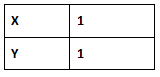A. (1, 1) is a solution of 2x + 5y = 7
B. If positive real numbers are chosen, 2x + 5y = 7 will have many solutions.
C. If real numbers are chosen, 2x + 5y = 7 will have infinite solutions.
D. If rational numbers are chosen, 2x + 5y = 7 will have many solutions.
Hence, option A is the correct answer.

Q.3. If (2, 0) is a solution of the linear equation 2x + 3y = k, then the value of k is
A. 4
B. 6
C. 5
D. 2
Ans
: A
Explanation:
We know that,
(2, 0) = (x, y)
Substituting values of x and y in the above equation, we get
2×2 + 3×0 = k
k = 4
Hence, option A is the correct answer.

Q.4. Any solution of the linear equation 2x + 0y + 9 = 0 in two variables is of the form
A. (-9/2 , m)
B. (n, -9/2)
C. (0, -9/2)
D. ( – 9, 0)
Ans
: A
Explanation:
Solving the above equation we get,
2x = -9
x = -9/2
As the coefficient of y is 0, therefore, y can take any value and will not affect our answer.
A. x = -9/2
y = any value
B. x = n
C. x = 0
D. x = -9
Hence, option A is the correct answer.

Q.5. The graph of the linear equation 2x + 3y = 6 cuts the y – axis at the point
A. (2, 0)
B. (0, 3)
C. (3, 0)
D. (0, 2)
Ans:
D
Explanation:
Let 2x + 3y = 6 cut the y-axis at P. therefore at P x-coordinate = 0.
Substituting x = 0, we get
2(0) + 3y = 6
3y = 6
y = 2
Hence the coordinates are (0, 2).
A. (2, 0) is wrong because it has x = 2
B. (0, 3) is wrong because it has y = 3
C. (3, 0) is wrong because it has x = 3
D. (0, 2) is right because it has x = 0 and y = 2 which is equal to the coordinates (0,2)
Hence, option D is the correct answer.

Q.6. The equation x = 7, in two variables, can be written as
A. 1. x + 1. y = 7
B. 1. x + 0. y = 7
C. 0. x + 1. y = 7
D. 0. x + 0. y = 7
Ans:
B
Explanation:
A. Simplifying the equation we get x + y = 7
B. Simplifying the equation we get x + 0y = 7 which is equal to x = 7
C. Simplifying the equation we get y = 7
D. simplifying the equation we get 0x + 0y = 7 which is not possible.
Hence, option (B) is the correct answer.

Q.7. Any point on the x – axis is of the form
A. (x, y)
B. (0, y)
C. (x, 0)
D. (x, x)
Ans: C

Explanation:
Any point on the x-axis has its ordinate 0.
So, any point on the x-axis if of the form (x, 0).
Hence, option (C) is the correct answer.

Q.8. Any point on the line y = x is of the form
A. (a, a)
B. (0, a)
C. (a, 0)
D. (a, – a)
Ans:
A
Explanation:
Any point on the line y = x will have x and y coordinate same.
So, any point on the line y = x is of the form (a, a).
Hence, option (A) is the correct answer.

Q.9. The equation of x – axis is of the form
A. x = 0
B. y = 0
C. x + y = 0
D. x = y
Ans:
B
Explanation:
The equation of x-axis is y = 0, since, x-axis is a parallel to itself at a distance 0 from it.
Hence, option (B) is the correct answer.

Exercise 4.2

Write whether the following statements are True or False? Justify your answers:

Q.1. The point (0, 3) lies on the graph of the linear equation 3x + 4y = 12.
Ans:
True.
Justification:
We have the equation, 3x + 4y = 12.
Substituting the values of x = 0 and y = 3 from the point (0, 3) in the equation,
We get,
3(0) + 4(3) = 12 = RHS.
Hence, the point (0, 3) lies on the graph of the linear equation 3x + 4y = 12.

Q.2. The graph of the linear equation x + 2y = 7 passes through the point (0, 7).

Ans:
False.
Justification:
We have the equation, x + 2y = 7.
Substituting the values of x = 0 and y = 7 from the point (0, 7) in the equation,
We get,
0 + 2(7) = 14 ≠RHS
Hence, the graph of the linear equation x + 2y = 7 passes through the point (0, 7).

Q.3. The graph given below represents the linear equation x + y = 0.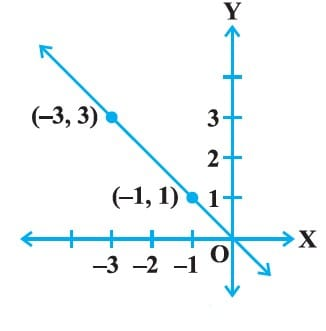Ans:
True.
Justification:
We have the equation, x + y = 0.
x + y = 0
x = – y
from the graph, we get the points ( – 3, 3) and ( – 1, 1), Considering the point ( – 3, 3)
x = – 3 and y = 3
Hence, substituting ( – 3, 3) in equation,
We get,
– 3 = 3 which satisfies the equation x = – y
Considering the point ( – 1, 1)
x = – 1 and y = 1
Hence, substituting ( – 1, 1) in equation,
We get,
– 1 = 1 which satisfies the equation x = – y
Therefore, the given solution: ( – 3, 3) and ( – 1, 1) satisfies the given equation x = – y.
Hence, the given graph represents the linear equation x + y = 0.

Exercise 4.3

Q.1. Draw the graphs of linear equations y = x and y = – x on the same Cartesian plane. What do you observe?
Ans:

According to the question,
y = x  ..... eq (i)
Values of x and y satisfying the equation=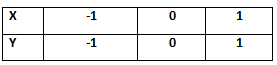y = -x ..... eq (ii)

Values of x and y satisfying the equation=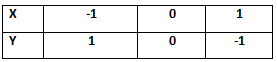Plotting the graph: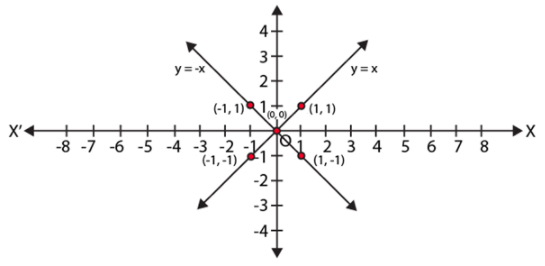From the above graph,

We observe that the two lines y = x and y = – x intersect each other at O (0, 0).

Q.2. Determine the point on the graph of the linear equation 2x + 5y = 19 whose ordinate is 1½ times its abscissa.
Ans:
From the question, we have,
2x + 5y = 19 …(i)
According to the question,
Ordinate is 1½ times its abscissa
⇒ y = 1½ x = (3/2) x
Substituting y = (3/2)x in eq. (i)
We get,

2x + 5 (3/2) x = 19
(19/2)x = 19
x = 2
Substituting x = 2 in eq. (i)
We get
2x + 5y = 19
2(2) + 5y = 19
y = (19 – 4)/5 = 3
Hence, we get x =2 and y = 3
Thus, point (2, 3) is the required solution.

Q.3. Draw the graph of the equation represented by a straight line which is parallel to the x-axis and at 3 units below.

Ans:
According to the question,
We get the linear equation,
y = – 3
Values of x and y satisfying the equation=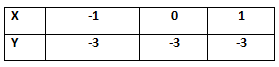Plotting the graph: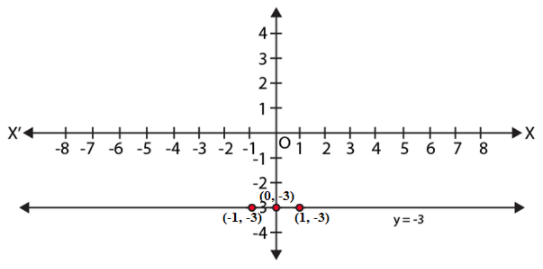Q.4. Draw the graph of the linear equation whose solutions are represented by the points having the sum of the coordinates as 10 units.

Ans:
According to the question,
We get the linear equation,

x + y = 10

We get,

x = 10 – y

Values of x and y satisfying the equation=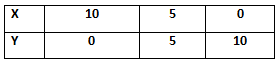Plotting the graph: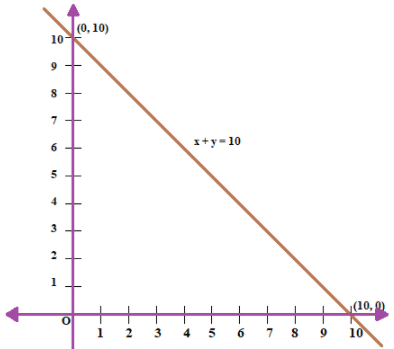Q.5. Write the linear equation such that each point on its graph has an ordinate 3 times its abscissa.
Ans:
According to the question,
A linear equation such that each point on its graph has an ordinate(y) which is 3 times its
abscissa(x).
So we get,
⇒ y = 3x.
Hence, y = 3x is the required linear equation.

Exercise 4.4

Q.1. Show that the points A (1, 2), B ( – 1, – 16) and C (0, – 7) lie on the graph of the linear equation y = 9x – 7.
Ans:

We have the equation,
y = 9x – 7
For A (1, 2),
Substituting the values of (x, y) = (1, 2),
We get,
2 = 9(1) – 7 = 9 – 7 = 2
For B (–1, –16),
Substituting the values of (x, y) = (–1, –16),
We get,
–16 = 9(–1) – 7 = – 9 – 7 = – 16
For C (0, –7),
Substituting the values of (x, y) = (0, –7),
We get,
– 7 = 9(0) – 7 = 0 – 7 = – 7
Hence, we find that the points A (1, 2), B (–1, –16) and C (0, –7) satisfies the line y = 9x – 7.
Hence, A (1, 2), B (–1, –16) and C (0, –7) are solutions of the linear equation y = 9x – 7
Therefore, points A (1, 2), B (–1, –16), C (0, –7) lie on the graph of linear equation y = 9x – 7.

Q.2. The following observed value of x and y are thought to satisfy a linear equation. Write the linear equation-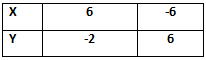Draw the graph using the value of x, y as given in the above table. At what points the graph of the linear equation (i) cuts the X-axis ? (ii) cuts the Y-axis?

Ans: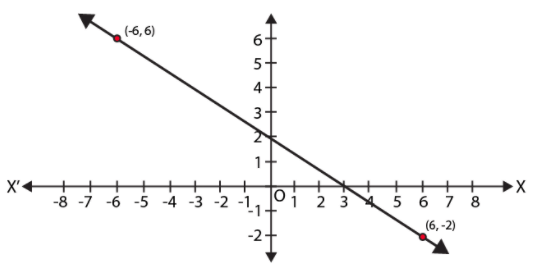We know that,
The linear equation of a line is,
y = mx + c, where, c is the y-intercept
From the graph,
We get y-intercept is 2.

⇒ c = 2.

Also, from the graph,

We get,
x1 = 6, y1 = – 2 and x2 = – 6, y2 = 6
We know that,
m = slope of the line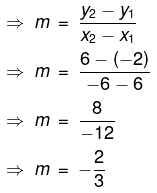∴ we get the linear equation,
y = – (2/3)x + 2
Multiplying whole equation by 3, we get,
⇒ 3y = – 2x + 6
⇒ 2x + 3y – 6 = 0
Thus, the points the graph of the linear equation cuts
(i) x-axis
Since, the point is on x axis, we have, y = 0.
Substituting y = 0 in the equation, 2x + 3y – 6 = 0,
We get,
2x + 3×0 – 6 = 0
⇒ 2x = 6
⇒ x = 3
Hence, the point at which the graph cuts x-axis = (3, 0).
(ii) y-axis
Since, the point is on y axis, we have, x = 0.
Substituting x = 0 in the equation, 2x + 3y – 6 = 0,
We get,
2×0 + 3y – 6 = 0
⇒ 3y = 6
⇒ y = 2
Hence, the point at which the graph cuts x-axis = (0, 2).

Q.3. Draw the graph of the linear equation 3x + 4y = 6. At what points, the graph cuts X and Y-axis?

Ans:

According to the question,
We get the equation,
3x + 4y = 6.
We need at least 2 points on the graph to draw the graph of this equation,
Thus, the points the graph cuts
(i) x-axis
Since, the point is on x axis, we have, y = 0.
Substituting y = 0 in the equation, 3x + 4y = 6,
We get,
3x + 4×0 = 6
⇒ 3x = 6
⇒ x = 2
Hence, the point at which the graph cuts x-axis = (2, 0).
(ii) y-axis
Since, the point is on y axis, we have, x = 0.
Substituting x = 0 in the equation, 3x + 4y = 6,
We get,
3×0 + 4y = 6
⇒ 4y = 6
⇒ y = 6/4

⇒ y = 3/2
⇒ y = 1.5
Hence, the point at which the graph cuts x-axis = (0, 1.5).
Plotting the points (0, 1.5) and (2, 0) on the graph.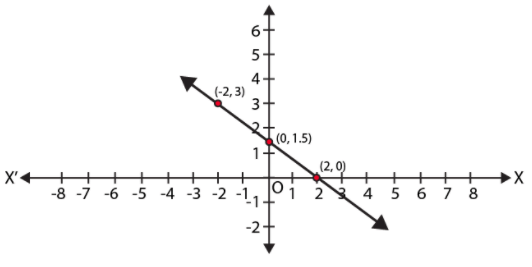The document NCERT Exemplar Solutions: Polynomials Notes | Study Mathematics (Maths) Class 9 - Class 9 is a part of the Class 9 Course Mathematics (Maths) Class 9.
All you need of Class 9 at this link: Class 9Use Code STAYHOME200 and get INR 200 additional OFF

## Mathematics (Maths) Class 9

73 videos|352 docs|109 tests

Track your progress, build streaks, highlight & save important lessons and more!

,

,

,

,

,

,

,

,

,

,

,

,

,

,

,

,

,

,

,

,

,

;